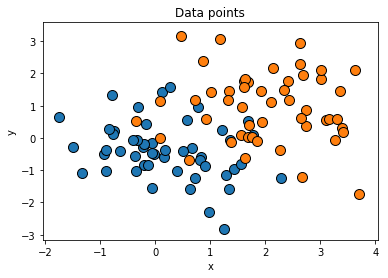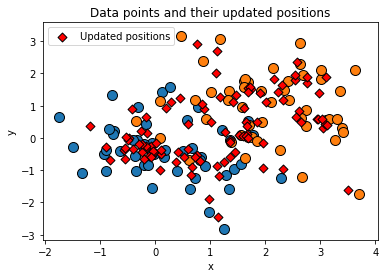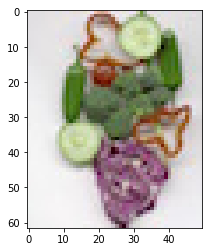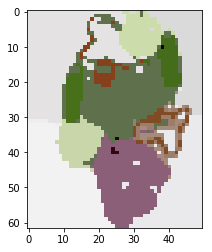# TP3 Mean Shift¶

Read, understand, and complete and run the following notebook. You must return the completed notebook, including your answers and illustrations (you may need to add cells to write your code or comments).

To execute a notebook, you will need to install jupyter. If you are using anaconda (strongly advised). If you cannot/don't want to use notebooks, you can return both your python code and a report in pdf. Note that all the TPs will use python2. If you are using python3, you might need to do a few changes to the provided code.

Return your work by e-mail using a single file (ipynb or zip) with the format introvis17_tp3_yourname.ipynb

# 0. Imports¶

In :
# /!\ I'm using Python 3 !

import numpy as np
# this is the key library for manipulating arrays. Use the online ressources! http://www.numpy.org/

import matplotlib.pyplot as plt
# used to read images, display and plot http://matplotlib.org/api/pyplot_api.html .
#You can also check this simple intro to using ipython notebook with images https://matplotlib.org/users/image_tutorial.html

%matplotlib inline
# to display directly in the notebook

import scipy.ndimage as ndimage
# one of several python libraries for image procession

plt.rcParams['image.cmap'] = 'gray'
# by default, the grayscale images are displayed with the jet colormap: use grayscale instead

from skimage.color import rgb2lab, lab2rgb
# for colorspace conversions

import plotly
import plotly.graph_objs as go

plotly.offline.init_notebook_mode(connected=True)

from IPython.core.display import display, HTML, Markdown
# The polling here is to ensure that plotly.js has already been loaded before
# setting display alignment in order to avoid a race condition.
display(HTML(
'<script>'
'var waitForPlotly = setInterval( function() {'
'if( typeof(window.Plotly) !== "undefined" ){'
'MathJax.Hub.Config({ SVG: { font: "STIX-Web" }, displayAlign: "center" });'
'MathJax.Hub.Queue(["setRenderer", MathJax.Hub, "SVG"]);'
'clearInterval(waitForPlotly);'
'}}, 250 );'
'</script>'
))


# 1. Mean shift¶

In this section, we will implement Mean Shift and test is on simple synthetic data.

## 1) Generate a random vector of 100 2D points data with 50 points sampled from a Gaussian distribution of variance 1 centered in (0,0) and 50 points sampled from a Gaussian distribution of variance 1 centered in (2,1). Plot your points using the plt.scatter function.¶

In :
# Generating data

n = 100 # number of points

# Means and Variances: works with any number of distributions to plot
mu = [np.array([0, 0]), np.array([2, 1])] # means of Gaussian distributions
sigma = [1, 1] # variances of Gaussian distributions

assert len(mu) == len(sigma)

k = len(mu) # number of distributions
X = [s * np.random.randn(n//k, 2) + m for m, s in zip(mu, sigma)]

# Plotting with Matplotlib

for x in X:
plt.scatter(x[:, 0], x[:, 1], s=100, edgecolors='black')

plt.title("Data points")
plt.xlabel("x")
plt.ylabel("y")
plt.show()

# Plotting with Plotly

ranges_x, ranges_y = [int(min(np.min(x) for x in X)-1), int(max(np.max(x) for x in X)+1)]

# Colorscale for data points
def colorscale_list(cmap, number_colors=k, return_rgb_only=False):
cm = plt.get_cmap(cmap)
colors = [np.array(cm(i/number_colors)) for i in range(1, number_colors+1)]
rgb_colors_plotly = []
rgb_colors_only = []
for i, c in enumerate(colors):
col = 'rgb{}'.format(tuple(255*c[:-1]))
rgb_colors_only.append(col)
rgb_colors_plotly.append([i/number_colors, col])
rgb_colors_plotly.append([(i+1)/number_colors, col])
return rgb_colors_only if return_rgb_only else rgb_colors_plotly

trace = go.Scatter(
x = np.concatenate(list(x[:, 0] for x in X)),
y = np.concatenate(list(x[:, 1] for x in X)),
mode = 'markers',
marker = dict(
size = 10,
color = [i for i in range(k) for _ in X[i]],
colorbar = dict(
title = 'Distributions',
titleside = 'top',
tickmode = 'array',
tickvals = list(range(k)),
ticktext = ['N({}, {})'.format(m, s) for m, s in zip(mu, sigma)]
),
showscale = True,
colorscale = colorscale_list('Set2'),
line = dict(
width = 2,
color = 'rgb(0, 0, 0)'
)
),
showlegend = False
)

def layout(title='Data points'):
return dict(
title = title,
xaxis = dict(
title = '$x$',
range = ranges_x,
ticklen = 5,
zeroline = False,
gridwidth = 2,
),
yaxis = dict(
title = '$y$',
range = ranges_y,
ticklen = 5,
gridwidth = 2,
),
legend = dict(
orientation = 'h',
y = -0.2
)
)

fig = dict(data=[trace], layout=layout())
plotly.offline.iplot(fig)## 2) We will segment images using a different distance parameter for space and color. For this reason, we will use a d dimensional vector sigma as a parameter for all our mean-shift functions. It defines a scale for each dimension. What would be a meaningful parameter for the synthetic data?¶

A value of $σ$:

• too small leads to overfitting
• too large leads to being unable to split the data points into different clusters

so there is a tradeoff to make.

In our case, as we know the Gaussian distributions that generate our data points, it might be reasonable to think that taking the mean of the standard deviations of each Gaussian respectively along x and along y (as we are in 2D), that is $σ = (1, 1)$ might be a good solution.

But as the two distributions overlap, in this case, $σ = (0.6, 0.6)$ is a better choice, to distinguish the two clusters (cf. question 5).

## 3) Implement the function MS_step which takes as input a vector of all the data point data, a starting point x and the standard deviations sigma and returns the updated position of the point x. Test it on your synthetic data.¶

In :
def MS_step(data, x, sigma):
d = sigma.shape # dimension
Sigma = np.diag(sigma)**2  # Covariance matrix
c = 1/np.sqrt(2 * np.pi * np.linalg.det(Sigma)) # K(X) = c exp(-(1/2) X^T Sigma^{-1} X)

SigmaInv = np.linalg.inv(Sigma) # Sigma^{-1}

def gaussian_exponent(differences):
# differences = x - (x_1 |... | x_n) = (x-x_1 |... | x-x_n)
# Sdiff = Sigma^{-1} (x-x_1 |... | x-x_n)
#       = (Sigma^{-1} (x-x_1) | ... | Sigma^{-1} x-x_n)
Sdiff = SigmaInv.dot(differences)

# computes -1/2 [(x-x_1)^T Sigma^{-1} (x-x_1), ..., (x-x_n)^T Sigma^{-1} (x-x_n)]
return -np.array([diff.dot(sdiff) for diff, sdiff in zip(differences.T, Sdiff.T)])/2

weights = c * np.exp(gaussian_exponent((x-data).T)) # [K(x-x_1), ..., K[x-x_n]]
return data.T.dot(weights)/np.sum(weights)

In :
data = np.vstack(X)
sigma = np.array([0.60, 0.60])

for x in X:
plt.scatter(x[:, 0], x[:, 1], s=100, edgecolors='black')

updated_pos = np.apply_along_axis(lambda x: MS_step(data, x, sigma), 1, data)

# With matplotlib

plt.scatter(updated_pos[:,0], updated_pos[:,1], s=40, edgecolors='black', color='r', marker="D", label="Updated positions")
plt.title("Data points and their updated positions")
plt.xlabel("x")
plt.ylabel("y")
plt.legend()
plt.show()

# With Plotly

trace_updated_1 = go.Scatter(
x = updated_pos[:, 0],
y = updated_pos[:, 1],
mode = 'markers',
marker = dict(
symbol = "star-diamond",
size = 7,
color = 'red',
line = dict(
width = 1,
color = 'rgb(0, 0, 0)'
)
),
name = 'MS: step 1'
)

fig = dict(data=[trace, trace_updated_1], layout=layout())
plotly.offline.iplot(fig)## 4) Implement the function MS_point which iterates MS_step until convergence (e.g. the estimate changes by less than 0.01). Test it on your synthetic data.¶

In :
def MS_point(data, x, sigma, return_list=False):
eps = 0.01
list_updated_pos = []

updated_pos = MS_step(data, x, sigma)
list_updated_pos.append(updated_pos)

# while the distance between x and the updated position is > eps,
# one continues to iterate MS_step
while np.linalg.norm(x - updated_pos) > eps:
x = updated_pos
updated_pos = MS_step(data, x, sigma)
list_updated_pos.append(updated_pos)

# return the list of all the updated positions or just the last one
return np.array(list_updated_pos) if return_list else updated_pos

In :
lists_updated_pos = [MS_point(data, x, sigma, return_list=True) for x in data]
list_by_step = []
max_iter = max(len(l) for l in lists_updated_pos)

for i in range(max_iter):
list_by_step.append(np.array([l[i] for l in lists_updated_pos if len(l)>i]))

# With Plotly
trace_updated = []
colors = colorscale_list('Reds', number_colors=int(1.5*len(list_by_step)), return_rgb_only=True)
legend_step = len(list_by_step)//5

for i, updated_pos in enumerate(list_by_step):
trace_updated.append(go.Scatter(
x = updated_pos[:, 0],
y = updated_pos[:, 1],
mode = 'markers',
hovertext = 'step {}'.format(i),
hoveron = "points",
marker = dict(
symbol = "star-diamond",
size = min(7+i//legend_step, 18),
color = colors[i],
line = dict(
width = 1,
color = 'rgb(0, 0, 0)'
)
),
name = 'MS: step {}'.format(i),
showlegend = (i % legend_step == 0)
))

fig = dict(data=[trace] + trace_updated, layout=layout())
plotly.offline.iplot(fig)


## 5) Implement the MS function, which implements the full mean shift algorithm by iterating MS_point on all the points, and merging modes which distance normalized by sigma -- sqrt[sum[((x-y)/sigma)**2) ]] -- is smaller than 0.5. It must return a list of the modes and a label (corresponding to a mode) for each point.¶

In :
def MS(data, sigma):
tolerance = 0.5
labels = np.zeros([data.shape,1])

# Computed the modes with repetitions
# and the indices at which the repetitions occur
modes_repeated = np.array([MS_point(data, x, sigma) for x in data])
axis=2) <= tolerance, 1).any(0)

# compute the labels corresponding to each mode
for ind, mode in enumerate(modes):
labels[np.linalg.norm((modes_repeated - mode)/sigma, axis=1) <= tolerance] = ind

return labels, modes

In :
for sigma in [np.array([0.7, 0.7]), np.array([0.6, 0.6]), np.array([0.5, 0.5])]:
labels, modes = MS(data, sigma)

number_labels = len(modes)

trace_labels = go.Scatter(
x = np.concatenate(list(x[:, 0] for x in X)),
y = np.concatenate(list(x[:, 1] for x in X)),
mode = 'markers',
marker = dict(
size = 12,
color = [i for i in range(k) for _ in X[i]],
colorbar = dict(
title = 'Distributions',
titleside = 'top',
tickmode = 'array',
tickvals = list(range(k)),
ticktext = ['N({}, {})'.format(m, s) for m, s in zip(mu, sigma)]
),
showscale = True,
colorscale = colorscale_list('Set2'),
line = dict(
width = 4,
color = labels.flatten(),
colorscale = colorscale_list('Paired', number_colors=number_labels),
)
),
showlegend = False
)

display(Markdown("### sigma = {}".format(sigma)))
fig = dict(data=[trace_labels], layout=layout(title='sigma = {}: Data points (fill colors) &\
Predicted labels (colors of the border)'.format(sigma)))
plotly.offline.iplot(fig)


# 2. Segmentation¶

## 1) Download this small image, load it and convert it to the Lab colorspace. Why is it necessary to change the colorspace? What are the range of the colorvalues in Lab?¶

In :
Lab_img = rgb2lab(plt.imread('legumes_small.jpg'))
print(Lab_img.shape)
plt.show()

(62, 50, 3)1. The Lab colorspace is best suited to match the visual appearance of the colors: that is, visually close colors are close in the Lab colorspace, which is what is expected for the segmentation.
2. Whereas in the RBG colorspace, it happens that visually identical colors have completely different rgb coordinates, which is problematic for segmentation purposes.

In the Lab colorspace (implemented in skimage):

• L ranges from 0 to 100
• a ranges from -127 to 128
• b ranges from -128 to 127.

## 2) In Mean Shift segmentation, all pixels in the image will be treated as data points including both their color and position in the image. Convert the (N,M,3) image into an (N,M,5) array incuding color and position information for each point. What would be a meaningful value for sigma?¶

Tip: use the np.meshgrid function

In :
xx, yy = np.meshgrid(np.arange(Lab_img.shape), np.arange(Lab_img.shape))

Lab_img_pos = np.concatenate((Lab_img, yy[:,:,None], xx[:,:,None]), axis=2)

data2 = np.reshape(Lab_img_pos, [-1, 5])
sigma2 = np.array([5,10,10,6,5])


For each data point $(L, a, b, x_1, x_2)$, as:

• $L ∈ ⟦0, 100⟧$
• $a ∈ ⟦-127, 128⟧$
• $b ∈ ⟦-128, 127⟧$
• $x_1 ∈ ⟦0, 61⟧$
• $x_2 ∈ ⟦0, 49⟧$

sigma = [10,20,20,6,5] seems to be a good compromise (approximately one twientieth (resp. tenth) of the range of Lab values (resp. x_1/x_2 values) for each coordinate).

## 3) Use the MS function from the previous section to compute a meaningful segmentation of the image. Visualize the results as an image by replacing the color values of the pixels associated to each mode by the color of the mode.¶

In :
import time
start = time.time()

labels, modes = MS(data2, sigma2)

end = time.time()
print('Time of execution: {:.2f}s'.format(end - start))

Time of execution: 267.40s

In :
# attribute to each label the color of the corresponding mode
labels_list = labels.tolist()

for ind, lab in enumerate(labels_list):
labels_list[ind] = [modes[int(lab)][:3]]

labels_colors = lab2rgb(np.reshape(np.array(labels_list), Lab_img.shape))

plt.imshow(labels_colors)

Out:
<matplotlib.image.AxesImage at 0x11e6d1c88>